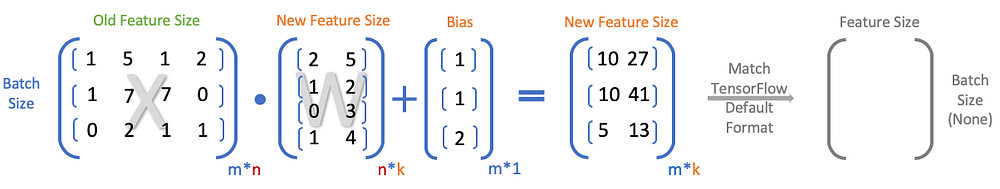# Mastering Linear Algebra in TensorFlow — Part1: Input Layer

Source: Deep Learning on Medium# Mastering Linear Algebra in TensorFlow — Part1: Tabular Data

One of the time-consuming issues for beginners is the matrix operations under different deep learning architectures. In other words, processing the linear algebra stuff represents how well you understand the mechanism of the matrix calculation. If you have little concept about matrix or tensor in linear algebra, checkout on Vihar Kurama’s post on Medium! To minimize confusion, TensorFlow 1.X and TensorFlow 2.X will be both included in this tutorial. All codes are executable on Google Colab for you can get into the topic in the shortest time.

# Major data formats

Before diving into detail In general, there are four major types of the input data format:

1. Vector Data

(1) Data shape: (data samples * features)

2. Timeseries Data or Sequence Data

(1) Data shape: (data samples * timestamps * features)

For sequence data, timestamps stand for sequence length of your input data, and the length should be fixed before feeding into the model. Usual methods include zero paddings and slicing. As for time series data, it represents each time period.

3. Image Data

(1) Data shape: (data samples * height * width * channels)

Height and width refer to the shape of the image. Channel represents things like RGB(then the channel is 3), greyscale(then the channel is 1), etc.

(2) Data shape: (data samples * features) if flattens height * width * channels

4. Video Data

(1) Data shape: (data samples * frame * height * width * channels)

(2) Data shape: (data samples * features) if flattens frame * height * width * channels

# Vector Data

Today we will focus on vector data and linear algebra handling when building this type of model. Note that data preprocessing may be ignored due to the things we are focusing on is not there this time. Vector data, also known as 2D tensors. If we say a single data is a vector, then 2D vector data is just when we handle more than one single data at a time. 2D vector data is the most common task in machine learning, and we sometimes transform high dimensional matrix such as image data into 2D vectors as well.

# Vector Data — Classification Problems

## 1. Data Preparation

Suppose we are implementing a 3-layers multilayer perceptron for the Iris dataset for classification on TensorFlow 1.X. First, we import some necessary packages:

On TensorFlow 1.X: